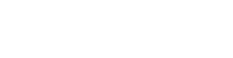# Physics Paper 3 Questions and Answers - Form 4 Term 1 Opener Exams 2021

PHYSICS
PAPER 3
(PRACTICAL)

FORM 4 TERM 1 OPENER EXAMS
TIME: 2½ HOURS

INSTRUCTIONS TO CANDIDATES

• Answer all the questions in the spaces provided.
• All workings must be clearly shown where necessary.
• Non- programmable silent electronic calculator may be used

Question 1

Part A
You are provided with the following apparatus

• A rectangular glass block
• Four optical pins
• Four office pins
• A plain white sheet of paper
• A soft board

Proceed as follows

1. Fix the plain sheet of paper on the soft board using the four office pins
2. Place the glass block on the plain sheet of paper and trace its outline. Remove the glass block and mark the outline MNOP.
3. Mark a point R on MN such that MR is a quarter of MN. Construct a perpendicular through R one edge MN through to edge PO
4. Draw line AR such that angle i = 10ᵒ and replace the glass block.
5. Fix pins P1 and P2 along AR and while viewing through the glass block from the opposite facing PO stick two other pins P3 and P4 in line with the image of P1 and P2. Remove the glass block and draw-line through P3 and P4 to meet PO at S. Join S to R (see figure below)6. Measure and record distance x and y on the table below
7. Repeat procedure (a) to (f) for values. Values of angle i = 20ᵒ, 30ᵒ, 40ᵒ, 50ᵒ, 60ᵒ and 70ᵒ and complete the table (8mks)
 Angle i X (cm) Y (cm) x/y Sin i 10ᵒ 20ᵒ 30ᵒ 40ᵒ 50ᵒ 60ᵒ 70ᵒ

1. On the grid provided plot a graph of sin i against x/y (5mks)
2. Determine the slope, n, of the graph (2mks)

Part B
You are provided with

• A cell and cell holder
• Switch
• A 10Ω carbon resistor labeled R
• Six connecting wires, two with a crocodile clip on one end
• An ammeter 0-1 A
• A voltmeter (0-5V) or (0-3V)

Procedure

1. Set up the apparatus as shown belowRecord the reading E of the voltmeter
E = …………………………….volts (1mk)
2. Close the switch and record the reading V of the voltmeter and I the reading of the ammeter
V……………………….. volts (1mk)
I……………………..Amperes (1mk)
3. Given that E=V+Ir, determine the value of r (2mks)

Question 2

Part A
You are provided with the following

• Water in a beaker
• Complete retort stand
• Two clamps
• 100ml measuring cylinder
• Boiling tube
• Meter rule
• Beam balance (can be shared)
• Vernier calipers (can be shared

Proceed as follows

1. Using the Vernier calipers, measure the internal diameter of the boiling tube D=…………………………………………. (1mk)
2.
1. Measure the length H, of the boiling tube
H=…………………………………….cm (1mk)
2. Measure the mass of the boiling tube using the beam balance M=…………………………………….g (1mk)
3. Clamp the boiling tube vertically with its base resting on a flat surface as shown, Use the second clamp to clamp the meter rule beside the boiling tube.4. Measure 10ml of water and pour into the boiling tube. Measure the height h, of the water. Keep adding water in small amounts in the boiling tube and complete the table below
 VOLUME IN CM3 ML HEIGHT H(CM) 10 20 35 45 50 65
5. On the grid provided, plot a graph of volume V(cm3) of water (y-axis) against height h (cm) (5mks)
6. From the graph determine the slope, (3mks)
7. Wind the cotton thread ten times round the boiling tube, pushing the windings very close together, the turns should not overlap on each other.L……………………… (cm) (1mk)
8. Calculate the volume V of the glass material which the boiling tube is made of, given that
V=h [2L2/2500−5]
V= ………………………………………. (2mks)
9. Calculate the density d in kg/m3, of the glass material of the boiling tube.
d=…………………………................... (3mks)

CONFIDENTIAL
Each candidate is required to have:

Questions 1

Part A

• A rectangular glass block
• Four office pins/masking tape/thumb pins
• Four optical pins
• A plain white sheet of paper
• A soft board

Part B

• A cell and cell holder
• Switch
• A 10 Ω carbon resistor labeled R
• Six connecting wires, two with 3 crocodile clips.
• An ammeter (0-1 A)
• A voltmeter (0-5V) or (0-3V)

Question 2

1. Complete retort stand with two clamps
2. Some water in a beaker (100ml)
3. 100ml measuring cylinder
4. Boiling tube
6. Meter rule
7. Beam balance (can be shared)
8. Vernier callipers (can be shared)

MARKING SCHEME

Question 1

Part A

 Angle i X (cm) Y (cm) x/y Sin i 10ᵒ 0.8 6.4 0.125 0.1736 20ᵒ 1.6 6.6 0.246 0.3420 30ᵒ 2.4 6.8 0.360 0.5000 40ᵒ 3.1 7.0 0.443 0.6428 50ᵒ 3.9 7.4 0.527 0.7660 60ᵒ 4.9 8.0 0.612 0.8660 70ᵒ 5.6 8.5 0.659 0.9397
1. Graph:2. Slope:
sin i
x/y
= 0.78 - 0.25
0.55 - 0.18
=0.53/0.37
=1.432

Part B

1. E= 1.6V ± 0.1
2. V= 1.4V ± 0.1
I= 0.1 ± 0.1
3. E= V+Ir
1.6=1.4 + 0.1r
r=2Ω

Question 2

1.    2.24cm ± 0.1
2.
1. H=14.9cm ± 0.1
2. 24.7g ± 1
3. .

4.  Volume of water in cm3/ml Height h(cm)(±0.8) 10 2.7 20 5.1 35 6.4 45 8.8 50 10.0 65 13.6
Each 1/2mk total 3mk

5. GridChange in y
Change in x
= 62-40
13-78
=225.2
=4.231cm(without unit ½mk)
7. L=86cm ± 1.0
8. H=(2L2/2500-5)= 14.9(2x(86)2/2500-4.231)
=14.9(14792/2500-4.231)
=149(5.9168 - 4.231)
= 14.9 x 1.6858 = 25.11842
9. Density = mass/ volume= 24.7/25.11842=0.9833g/cm3

• ✔ To read offline at any time.
• ✔ To Print at your convenience
• ✔ Share Easily with Friends / Students